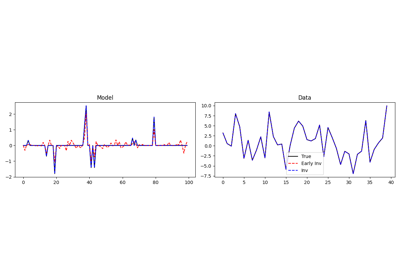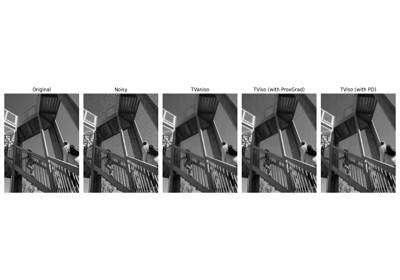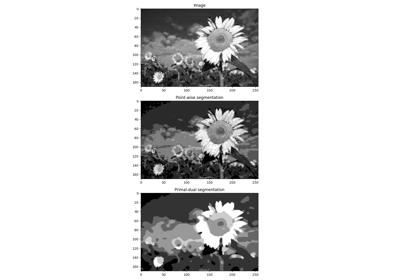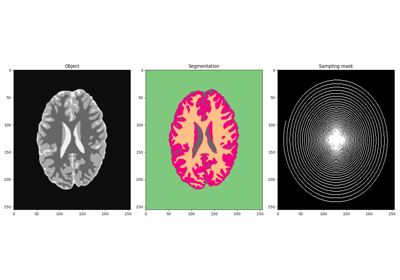# pyproximal.optimization.primaldual.PrimalDual#

pyproximal.optimization.primaldual.PrimalDual(proxf, proxg, A, x0, tau, mu, y0=None, z=None, theta=1.0, niter=10, gfirst=True, callback=None, callbacky=False, returny=False, show=False)[source]#

Primal-dual algorithm

Solves the following (possibly) nonlinear minimization problem using the general version of the first-order primal-dual algorithm of :

$\min_{\mathbf{x} \in X} g(\mathbf{Ax}) + f(\mathbf{x}) + \mathbf{z}^T \mathbf{x}$

where $$\mathbf{A}$$ is a linear operator, $$f$$ and $$g$$ can be any convex functions that have a known proximal operator.

This functional is effectively minimized by solving its equivalent primal-dual problem (primal in $$f$$, dual in $$g$$):

$\min_{\mathbf{x} \in X} \max_{\mathbf{y} \in Y} \mathbf{y}^T(\mathbf{Ax}) + \mathbf{z}^T \mathbf{x} + f(\mathbf{x}) - g^*(\mathbf{y})$

where $$\mathbf{y}$$ is the so-called dual variable.

Parameters
proxfpyproximal.ProxOperator

Proximal operator of f function

proxgpyproximal.ProxOperator

Proximal operator of g function

Apylops.LinearOperator

Linear operator of g

x0numpy.ndarray

Initial vector

taufloat or np.ndarray

Stepsize of subgradient of $$f$$. This can be constant or function of iterations (in the latter cases provided as np.ndarray)

mufloat or np.ndarray

Stepsize of subgradient of $$g^*$$. This can be constant or function of iterations (in the latter cases provided as np.ndarray)

z0numpy.ndarray

Initial auxiliary vector

znumpy.ndarray, optional

thetafloat

Scalar between 0 and 1 that defines the update of the $$\bar{\mathbf{x}}$$ variable - note that theta=0 is a special case that represents the semi-implicit classical Arrow-Hurwicz algorithm

niterint, optional

Number of iterations of iterative scheme

gfirstbool, optional

Apply Proximal of operator g first (True) or Proximal of operator f first (False)

callbackcallable, optional

Function with signature (callback(x)) to call after each iteration where x is the current model vector

callbackybool, optional

Modify callback signature to (callback(x, y)) when callbacky=True

returnybool, optional

Return also y

showbool, optional

Display iterations log

Returns
xnumpy.ndarray

Inverted model

Notes

The Primal-dual algorithm can be expressed by the following recursion (gfirst=True):

$\begin{split}\mathbf{y}^{k+1} = \prox_{\mu g^*}(\mathbf{y}^{k} + \mu \mathbf{A}\bar{\mathbf{x}}^{k})\\ \mathbf{x}^{k+1} = \prox_{\tau f}(\mathbf{x}^{k} - \tau (\mathbf{A}^H \mathbf{y}^{k+1} + \mathbf{z})) \\ \bar{\mathbf{x}}^{k+1} = \mathbf{x}^{k+1} + \theta (\mathbf{x}^{k+1} - \mathbf{x}^k)\end{split}$

where $$\tau \mu \lambda_{max}(\mathbf{A}^H\mathbf{A}) < 1$$.

Alternatively for gfirst=False the scheme becomes:

$\begin{split}\mathbf{x}^{k+1} = \prox_{\tau f}(\mathbf{x}^{k} - \tau (\mathbf{A}^H \mathbf{y}^{k} + \mathbf{z})) \\ \bar{\mathbf{x}}^{k+1} = \mathbf{x}^{k+1} + \theta (\mathbf{x}^{k+1} - \mathbf{x}^k) \\ \mathbf{y}^{k+1} = \prox_{\mu g^*}(\mathbf{y}^{k} + \mu \mathbf{A}\bar{\mathbf{x}}^{k+1})\end{split}$
1

A., Chambolle, and T., Pock, “A first-order primal-dual algorithm for convex problems with applications to imaging”, Journal of Mathematical Imaging and Vision, 40, 8pp. 120-145. 2011.

## Examples using pyproximal.optimization.primaldual.PrimalDual#Basis Pursuit

Basis PursuitDenoising

DenoisingImage segmentation

Image segmentationMRI Imaging and Segmentation of Brain

MRI Imaging and Segmentation of Brain